# Division Sentences

Division Sentences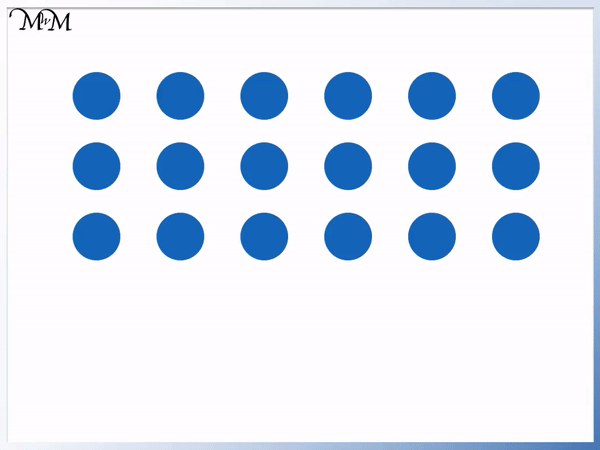• A division sentence is a mathematical way to show how an amount is shared.
• Here we have 18 counters in total.
• We share the counters into 6 equal groups.
• There are 3 counters in every group.
• We write the total amount first, which is 18.
• The division sign, ÷, means to share the amount before it by the amount after it.
• 6 is the number of groups, so we write a 6 after the division sign.
• There are 3 counters in each group so we write a 3 after the equals sign, =.
• 18 ÷ 6 = 3 means that 18 shared into 6 equal groups gives us 3 in every group.

The larger number is written before the division sign.

The number of groups comes after the division sign and the number in each group comes after the equals sign.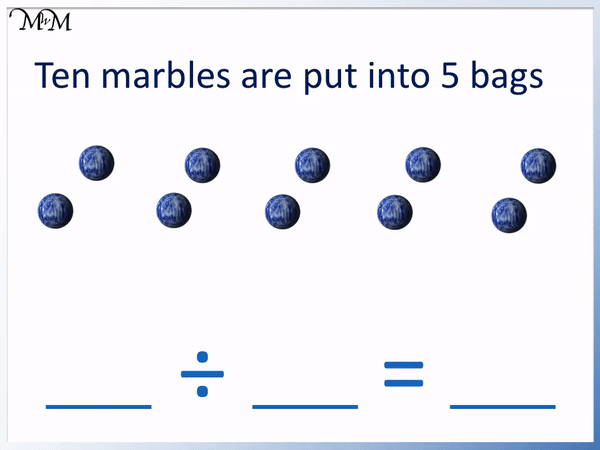• Ten marbles are put into 5 bags.
• The total number of marbles is 10 and so, we write this number first.
• We then write the division sign, ÷.
• We are sharing the marbles into 5 parts and so, we write 5 after the division sign.
• 10 marbles shared into 5 is written as 10 ÷ 5.
• We then write an equals sign followed by the number of marbles in each bag.
• There are 2 marbles in each bag.
• 10 ÷ 5 = 2 means that 10 shared into 5 equal parts gives us 2 in each group.
• The division sentence is 10 ÷ 5 = 2.Supporting Lessons# Division Sentences

## What is a Division Sentence?

A division sentence is made up of 3 numbers, a division sign and an equals sign. The first number, before the division sign, tells us the total amount being shared. The second number, after the division sign, tells us how many groups we are sharing the amount into. The third number, after the equals sign, tells us the number in each group after the division.

20 ÷ 5 = 4 is an example of a division sentence. It contains three numbers, a division sign and an equals sign.

20 ÷ 5 = 4 means that 20 shared into 5 equal groups would give us 4 in each group.

We can teach division sentences using arrays of counters. We can circle groups of counters to group them.

Below we have 20 counters.We share the counters into 5 equal groups. This means that we must have the same number of counters in each group.

There are 4 counters in each of the 5 groups.

The division sentence 20 ÷ 5 = 4 represents this problem.The first number is 20, which is the total number of counters. We always write the total number of objects we are sharing first.

The second number is the number of groups we have. When teaching division sentences, we can circle the groups with a pencil, or if using real counters, we can put them into pots. We can then count the number of groups or pots.

The final number after the equals sign is the number in each group. We can count the number of counters in each circle. If we put the counters in each pot, we can count these.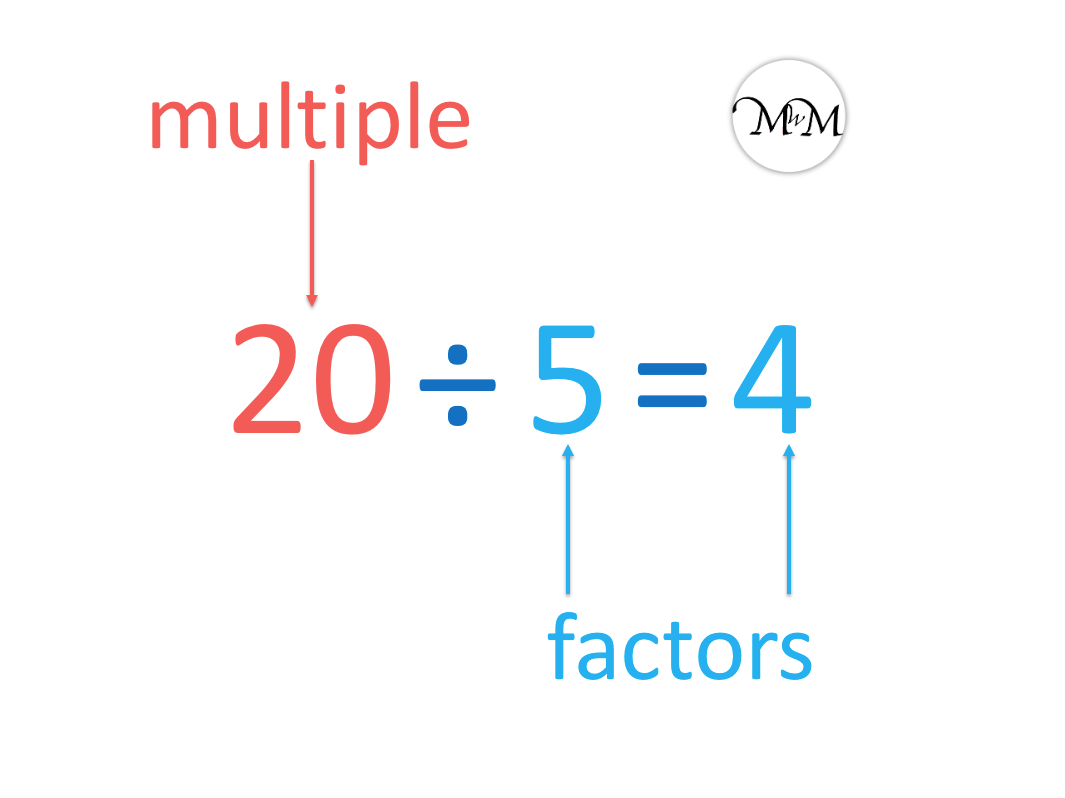There are 3 parts to a typical division sentence.

The first part is the number being shared. The second part is the number of groups the total is shared into. A division sign, ÷, separates the first and second parts.

The third part is the number in each group. An equals sign separates the second and third parts.

The first, largest number in a division is called the multiple.

The other two numbers in a division sentence are called factors. The two factors can be multiplied together to make the multiple.

Here is another example of a division sentence.

We have 18 ÷ 6 = 3.

This division sentence means that 18 shared into 6 equal groups gives us 3 in each group.

Again we will teach this division with counters. It is a good idea to introduce division to your child using physical models.18 is the total number and the largest number. This will go first in our number sentence.

The number of groups is 6.

The number of counters in each group is 3.

When teaching writing division sentences, it is useful to use physical counters and write the division sentence as you go. We can count the number of counters in total as the first step before writing this number down. We can share the counters equally into pots and count the number of pots. The final number is the number of counters in each pot.

Following these steps keeps the numbers in the division sentence in the correct order.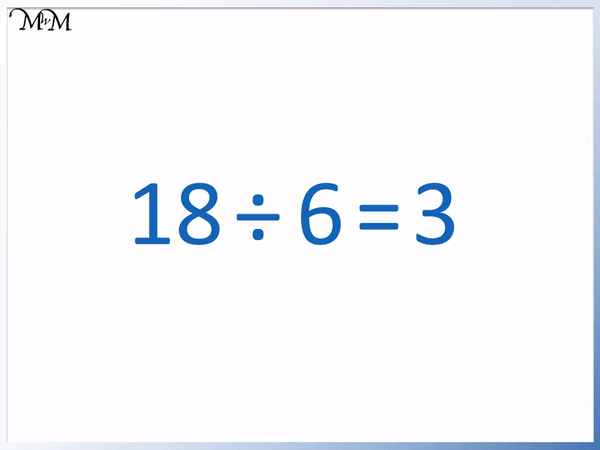The number 20 is the multiple and the smaller numbers, 5 and 4 are the factors.

The two factors can multiply together to make the multiple.## How to Write a Division Sentence

To write a division sentence use the following steps:

1. Write down the total number being shared or divided first.
2. Next write the division sign, ÷.
3. After the division sign, write down the number of groups the amount is being shared into.
4. Next write the equals sign, =.
5. Finally, write the number in each group after the objects have been shared.

As long as we use whole numbers, the largest number in a division sentence will come first.

Here is an example of writing a division sentence for a word problem.

Ten marbles are put into 5 bags.The first step is to write the total number being shared, which is 10.

The second step is to write a division sign, ÷.

The third step is to write the amount of groups. This is the number of bags the marbles will be put into. We have 5.

The fourth step is two write an equals sign, =.

The fifth step is to write the number in each group. By placing the marbles into 5 equal groups we can see that there are 5 in each group.

The division sentence is 10 ÷ 5 = 2.

This sentence means that 10 shared into 5 equal groups gives us 2 in each group.

Here is another example of writing a division sentence for a word problem.

12 apples are shared between 4 children.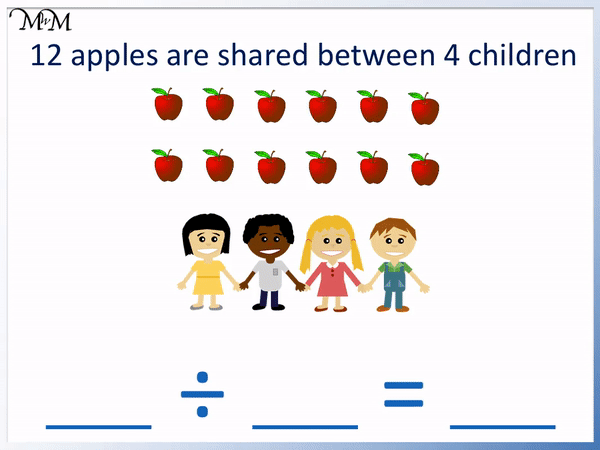Remember that the largest number in the division sentence will come first.

We are sharing 12 objects between 4 people.

We write 12 ÷ 4.

Each child gets 3 and so, our answer to the division is 3.

12 ÷ 4 = 3 means that 12 apples shared between 4 children gives each child 3 apples.

Here is an example of writing a division.

16 birds are put into groups of 4.The largest number is the total. We have 16 birds so we write this first.

The number after the division sign is the number of groups.

We have 16 ÷ 4, which means 16 birds shared into 4 equal groups.

We count how many birds in each group to get our answer, after the equals sign.

There are 4 birds in each group and so, 16 ÷ 4 = 4.Now try our lesson on Short Division without Remainders where we learn how to use the short division method for dividing numbers.error: Content is protected !!